• ## MATLAB中神经网络工具箱的使用

万次阅读 多人点赞 2017-03-24 19:20:18
今夕何夕兮，前些天把玩了一下MATLAB中神经网络工具箱的使用，忽有“扪参历井仰胁息”之感。别的倒是没什么，只是神经网络的数据组织结构有些“怪异”，要是不小心就会导致工具箱报错。以下便是神经网络工具箱的正确...
今夕何夕兮，前些天把玩了一下MATLAB中神经网络工具箱的使用，忽有“扪参历井仰胁息”之感。别的倒是没什么，只是神经网络的数据组织结构有些“怪异”，要是不小心就会导致工具箱报错。以下便是神经网络工具箱的正确打开姿势，谨供诸君参考：

1.打开MATLAB，在命令行输入nntool，将出现如下界面：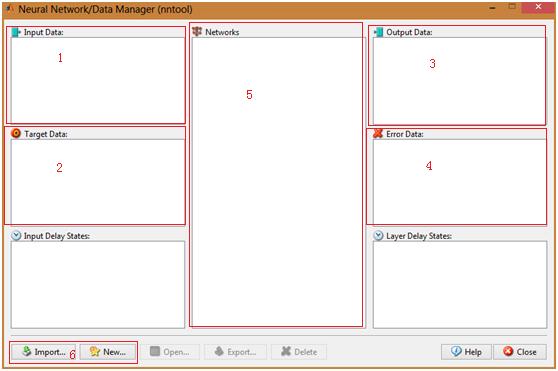图1 神经网络工具箱主界面

其中最主要的分为6个部分：第1部分中显示的是系统的输入数据；第2部分是系统的期望输出；第3部分是网络的计算输出；第4部分是网络的误差，即2和3之间的差异；第5部分呈现的是已经建立的神经网络实例；第6部分的两个按钮分别负责数据的导入和网络模型的建立。

2.点击“Import”按钮，分别导入输入数据与目标输出数据（数据可从工作区导入，也可从文件导入）：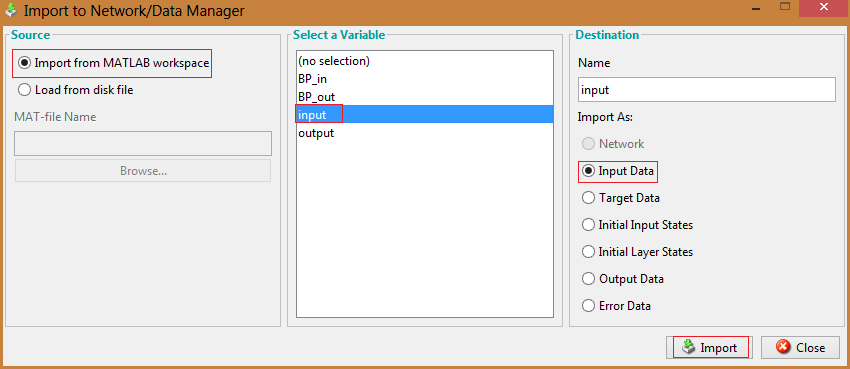图2 导入输入数据集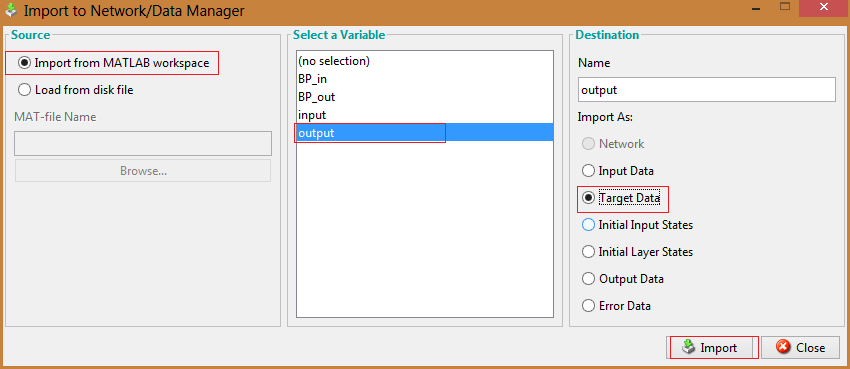图3 导入期望输出数据集

导入数据后主界面的情况如下：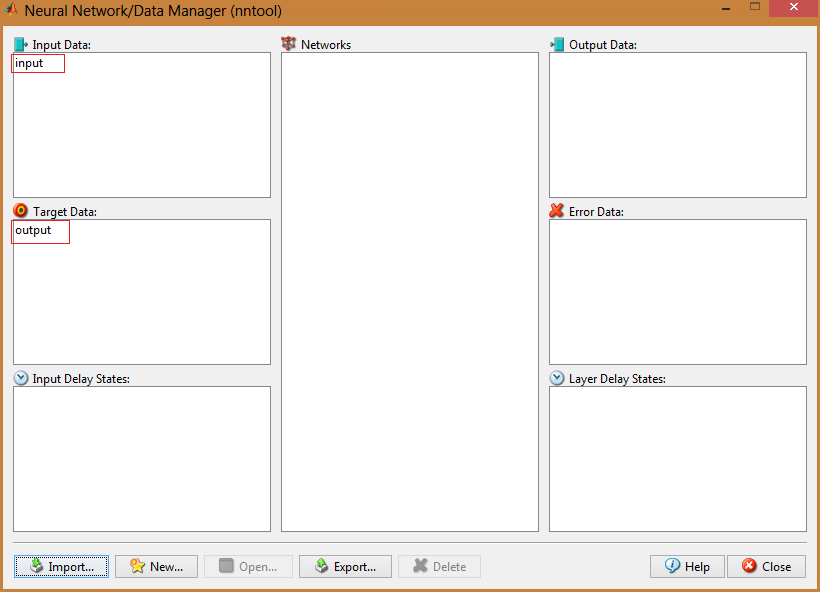图4 导入数据后的情况

重要说明：神经网络的数据是以列为基本单位的，即输入与输出数据的列数必须相同，否则将报错！如果原先数据是以行为单位组织的话，可以先在MATLAB中实现转置然后再导入，即B = A’。

3.现在需要的数据已经有了，下一步就是建立一个神经网络模型对数据集进行学习。以下步骤以BP网络为例，首先点击“New”按钮，出现如下界面：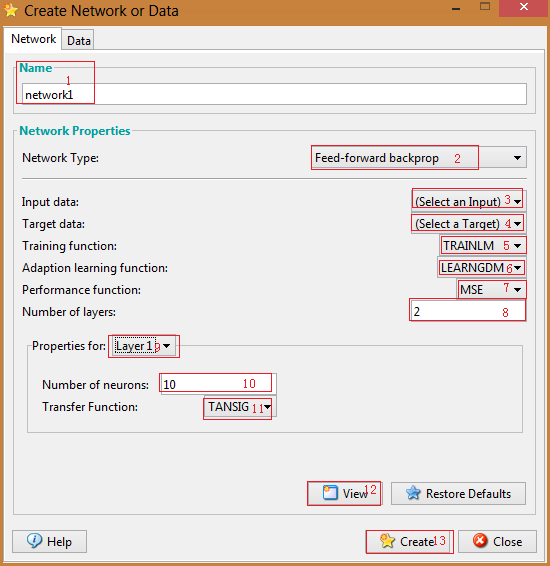图5 神经网络模型设置

几个重要部分已在上图中框出：1处用于定义该神经网络的名称；2处用于选择神经网络的类型；3处用于选择网络的输入数据；4处用于确定网络的期望输出数据；5、6、7处分别对神经网络的主要机制函数进行设置；8处设置网络层数；9处用于选择各网络层（需要说明的是：第1层指的是隐含层而不是输入层），从而在10和11处可以对该层的神经元个数和传递函数进行设置；12处按钮可以用于查看当前所设置的神经网络的结构图（下附图）；点击13处按钮即可生成对应的神经网络模型。前面只是简单地介绍了各个部分的作用，具体参数应该如何设置就只有各位自行去学习相关的文献了，此处不再多言。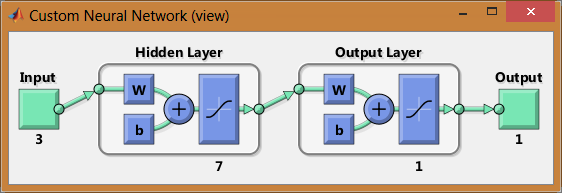图6 神经网络结构预览

4.现在模型和数据都有了，下一步该进行模型的训练了。回到主界面如下：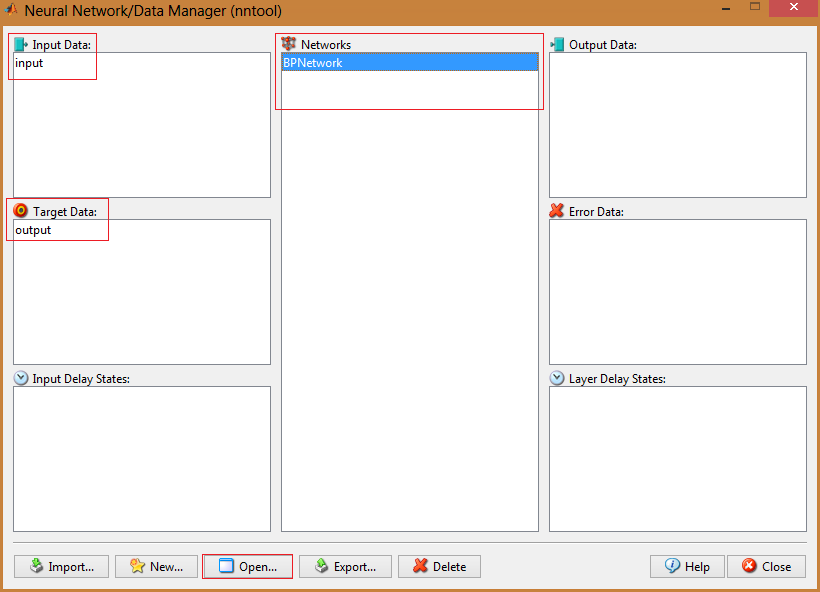图7 回到主界面

选中我们刚才建立的神经网络模型，然后点击“Open”按钮，将会出现如下界面：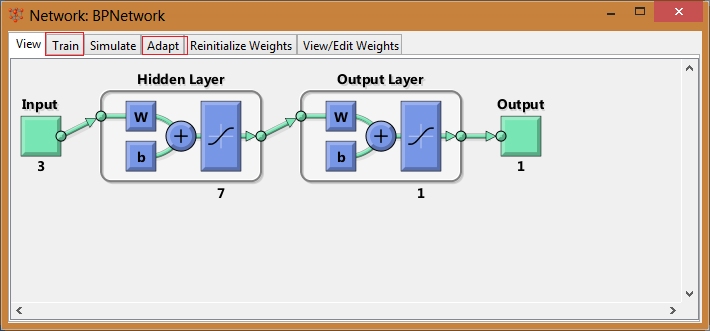图8 神经网络界面

在这里主要介绍两个选项卡中的内容，一个是“Train”，另一个是“Adapt”。点击“Train”选项卡后做相应的设置即可进行神经网络的训练：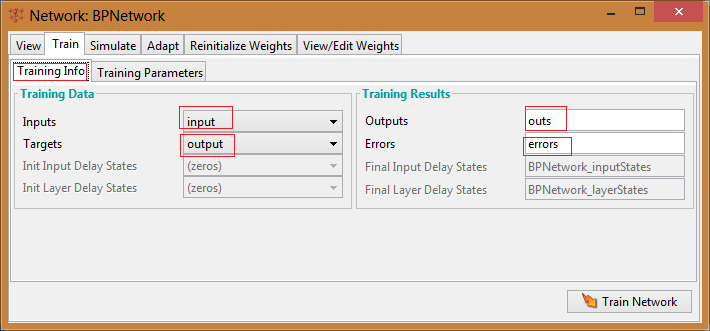图9 模型主要信息设置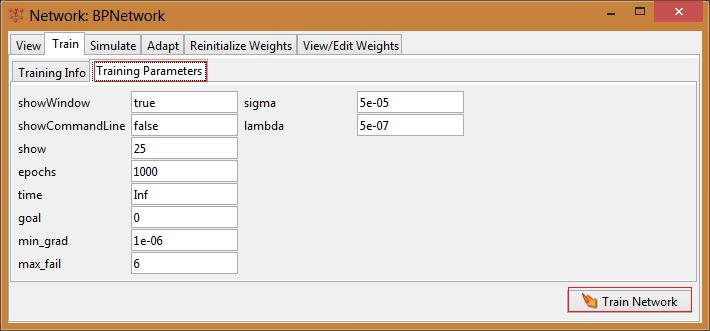图10 模型具体参数设置

设置完所有信息后点击“TrainNetwork”按钮即可进行网络的训练了。训练完成后会有一个结果信息界面，如下：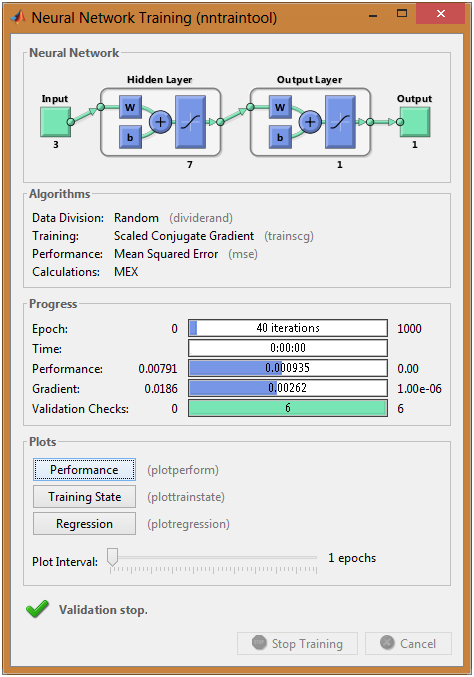图11 训练结果反馈

5.OK，现在模型训练也结束了，那么下一步自然是要来验证我们训练的模型。先导入验证输入和验证输出，这一步不再重提。然后来到模型验证界面：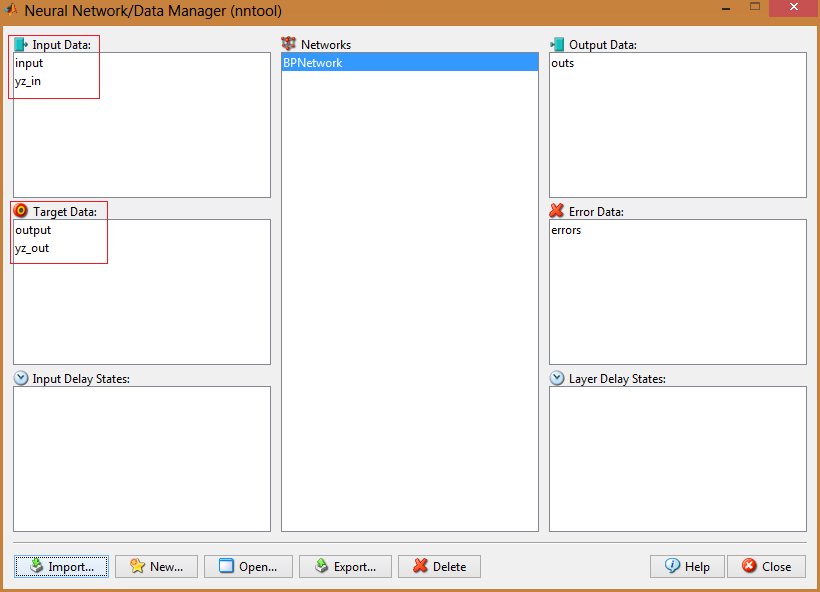图12 验证数据导入后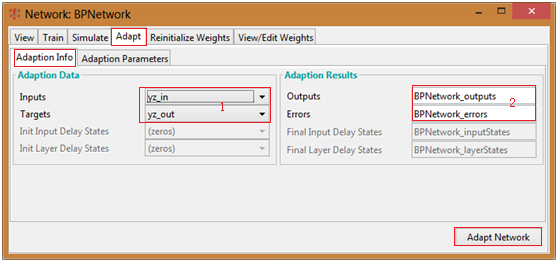图13 验证参数设置

红框1中设置网络的输入和验证输出；2中设置网络输出和误差情况的存储名；这些都完成之后点击“Adapt Network”后即可。此后会出现如下的提示界面：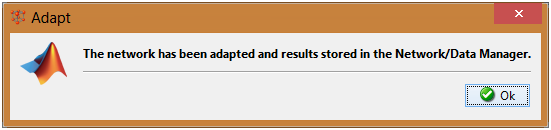图 14 提示界面

接下来再回到神经网络主界面如下：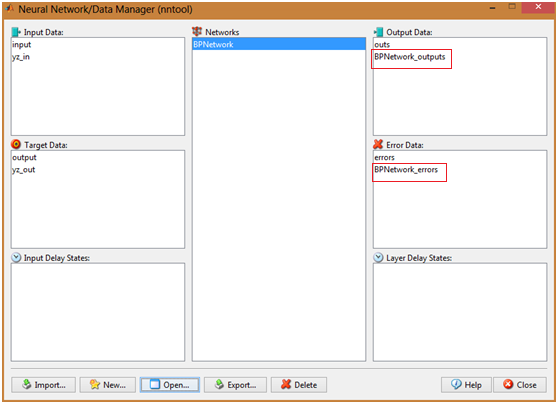图15 网络验证结果

此时界面中会多出红框所框出的两组数据，它们分别是网络的输出与对应的输出误差。具体的数据可以通过双击它们来打开查看。

重要说明：

神经网络的输入和输出数据要求每列为一个样本，按平常的习惯可能需要转置一下。

否则可能会报输入/输出样本数量不同的错误。

如果出现“input data size does not match net.inputs{1}.size”错误，那是因为创建神经网络是设定的输入个数与样本数据的输入个数不同而引起的，重新创建合适的神经网络即可。


展开全文• java调用matlab中神经网络工具箱编程一、安装matlab、java，配置好java环境应为matlab生成jar时直接调用的命令行，所以配置好java的标准就是在cmd里java和javac命令都可以用了~matlab据说低版本的也不能导出，所以就...
java调用matlab中神经网络工具箱编程一、安装matlab、java，配置好java环境应为matlab生成jar时直接调用的命令行，所以配置好java的标准就是在cmd里java和javac命令都可以用了~matlab据说低版本的也不能导出，所以就选个新点儿吧，我的是2010a二、在matlab中编写function文件三、运行matlab中的deployment tool，新建一个项目，选择target一定是java的哦~四、添加类，编辑类名五、添加刚刚的function文件六、编译~至此算是完成了，编译完之后再distrib文件夹下会有一个以你项目命名的jar文件，将这个文件和javabuilder.jar()添加到java程序的运行环境中就可以了，调用方法：// 训练好的net文件，从matlab中导出的String matStr = "C:/matlab.mat";MWCharArray matPath = new MWCharArray(matStr);// 要进行test的数据double[][] xn_test = { { 71.60 }, { 0.00 }, { 107.41 }, { 110.99 }, { 0.00 } };MWNumericArray test = new MWNumericArray(xn_test, MWClassID.DOUBLE);// 声明打包的类，调用测试方法Net_RBF netRBF = new Net_RBF();Object[] result = netRBF.rbfTest(1, matPath, test);//这个1是指输出结果的数目，具体看distrib下的doc// 输出结果System.out.println("计算结果是：" + result + "\t");推荐一篇文章：这个写的详细些，就是看不太清，呵呵http://www.doczj.com/doc/6bad0d0dec3a87c24128c42c.html/p-55963408.html遇到的错误：一、Attempt to use an MWArray that has been disposed用错对象了，本来是要用new MWCharArray(str);，结果用成了new MWNumericArray(str, MWClassID.CHAR)二、undefined function or method 'newrb' for input arguments of type 'double”先说一堆解释：
展开全文• 新版Matlab中神经网络训练函数Newff的详细讲解-新版Matlab中神经网络训练函数Newff的使用方法.doc 本帖最后由 小小2008鸟 于 2013-1-15 21:42 编辑 新版Matlab中神经网络训练函数Newff的详细讲解 一、 ...
• matlab中神经网络的运用，有详细介绍在matlab中神经网络的运用，有详细介绍在matlab中神经网络的运用，有详细介绍
• 我正在尝试使用matlabpython引擎matlab神经网络工具箱。在net = fitnet(10);net = train(net,x,t);view(net)y = net(x);perf = perform(net,y,t)然后我有以下python代码：^{pr2}$当我运行eng.net = eng.fitnet... 我正在尝试使用matlabpython引擎中的matlab神经网络工具箱。在net = fitnet(10);net = train(net,x,t);view(net)y = net(x);perf = perform(net,y,t)然后我有以下python代码：^{pr2}$当我运行eng.net = eng.fitnet(10.);行时我得到以下错误：In : ---------------------------------------------------------------------------TypeError                                 Traceback (most recent call last) in ()----> 1 eng.net = eng.fitnet(10.);/home/donbeo/myApp/matlab_python_engine/installdir/lib/python3.4/site-packages/matlab/engine/matlabengine.py in __call__(self, *args, **kwargs)77         else:78             return FutureResult(self._engine(), future, nargs, _stdout,---> 79                                 _stderr).result()8081     def __validate_engine(self):/home/donbeo/myApp/matlab_python_engine/installdir/lib/python3.4/site-packages/matlab/engine/futureresult.py in result(self, timeout)105106             self._result = pythonengine.getFEvalResult(--> 107                 self._future,self._nargout, None, out=self._out, err=self._err)108             self._retrieved = True109             return self._resultTypeError: Unsupported datatype returned from MATLAB.In :我怎么解决？在编辑：命令eng.net = eng.fitnet(10);退货：Error using network/subsasgn>network_subsasgn (line 553)"layers{1}.size" must be a positive integer.Error in network/subsasgn (line 13)net = network_subsasgn(net,subscripts,v,netname);Error in feedforwardnet>create_network (line 116)net.layers{i}.size = param.hiddenSizes(i);Error in feedforwardnet (line 69)net = create_network(param);Error in fitnet>create_network (line 98)net = feedforwardnet(param.hiddenSizes,param.trainFcn);Error in fitnet (line 70)net = create_network(param);---------------------------------------------------------------------------MatlabExecutionError                      Traceback (most recent call last) in ()----> 1 eng.net = eng.fitnet(10);/home/donbeo/myApp/matlab_python_engine/installdir/lib/python3.4/site-packages/matlab/engine/matlabengine.py in __call__(self, *args, **kwargs)77         else:78             return FutureResult(self._engine(), future, nargs, _stdout,---> 79                                 _stderr).result()8081     def __validate_engine(self):/home/donbeo/myApp/matlab_python_engine/installdir/lib/python3.4/site-packages/matlab/engine/futureresult.py in result(self, timeout)105106             self._result = pythonengine.getFEvalResult(--> 107                 self._future,self._nargout, None, out=self._out, err=self._err)108             self._retrieved = True109             return self._resultMatlabExecutionError:File /home/donbeo/myApp/MatlabR2015a/toolbox/nnet/nnet/@network/subsasgn.p, line 553, in network_subsasgnFile /home/donbeo/myApp/MatlabR2015a/toolbox/nnet/nnet/@network/subsasgn.p, line 13, in subsasgnFile /home/donbeo/myApp/MatlabR2015a/toolbox/nnet/nnet/nnnetwork/feedforwardnet.m, line 116, in create_networkFile /home/donbeo/myApp/MatlabR2015a/toolbox/nnet/nnet/nnnetwork/feedforwardnet.m, line 69, in feedforwardnetFile /home/donbeo/myApp/MatlabR2015a/toolbox/nnet/nnet/nnnetwork/fitnet.m, line 98, in create_networkFile /home/donbeo/myApp/MatlabR2015a/toolbox/nnet/nnet/nnnetwork/fitnet.m, line 70, in fitnet"layers{1}.size" must be a positive integer.In :
展开全文• 新版Matlab中神经网络训练函数Newff的使用方法一、 介绍新版newffSyntax· net = newff(P,T,[S1 S2...S(N-l)],{TF1 TF2...TFNl}, BTF,BLF,PF,IPF,OPF,DDF)Description newff(P,T,[S1 S2...S(N-l)],{TF1 TF2......
新版Matlab中神经网络训练函数Newff的使用方法一、   介绍新版newffSyntax·         net = newff(P,T,[S1 S2...S(N-l)],{TF1 TF2...TFNl}, BTF,BLF,PF,IPF,OPF,DDF)Description newff(P,T,[S1 S2...S(N-l)],{TF1 TF2...TFNl}, BTF,BLF,PF,IPF,OPF,DDF) takes several argumentsPR x Q1 matrix of Q1 sample R-element input vectorsTSN x Q2 matrix of Q2 sample SN-element target vectorsSiSize of ith layer, for N-1 layers, default = [ ].(Output layer size SN is determined from T.)TFiTransfer function of ith layer. (Default = 'tansig' forhidden layers and 'purelin' for output layer.)BTFBackpropagation network training function (default = 'trainlm')BLFBackpropagation weight/bias learning function (default = 'learngdm')IPFRow cell array of input processing functions. (Default = {'fixunknowns','removeconstantrows','mapminmax'})OPFRow cell array of output processing functions. (Default = {'removeconstantrows','mapminmax'})DDFData divison function (default = 'dividerand') ExamplesHere is a problem consisting of inputs P and targets T to be solved with a network.·         P = [0 1 2 3 4 5 6 7 8 9 10];T = [0 1 2 3 4 3 2 1 2 3 4];Here a network is created with one hidden layer of five neurons.·         net = newff(P,T,5);The network is simulated and its output plotted against the targets.·         Y = sim(net,P);plot(P,T,P,Y,'o')The network is trained for 50 epochs. Again the network's output is plotted.·         net.trainParam.epochs = 50;net = train(net,P,T);Y = sim(net,P);plot(P,T,P,Y,'o')二、   新版newff与旧版newff调用语法对比Example1比如输入input（6*1000），输出output为（4*1000），那么 旧版定义：net=newff(minmax(input),[14,4],{'tansig','purelin'},'trainlm');新版定义：net=newff(input,output,14,{'tansig','purelin'},'trainlm');Example2比如输入input（6*1000），输出output为（4*1000），那么 旧版定义：net=newff(minmax(input),[49,14,4],{'tansig','tansig','tansig'},'traingdx');新版定义：net=newff(input,output, [49,14], {'tansig','tansig','tansig'},'traingdx');  三、   旧版newff使用方法在新版本中使用提示：旧版本定义的newff虽也能在新版本中使用，但会有警告，警告如下： Warning: NEWFF used in an obsolete way.> In obs_use at 18  In newff>create_network at 127  In newff at 102          See help for NEWFF to update calls to the new argument list.四、   新版newff与旧版newff使用的训练效果对比     旧版本：旧用法训练次数多，但精度高  新版本：新用法训练次数少，但精度可能达不到要求造成上述原因是：程序里面的权值、阈值的初始值是随机赋值的，所以每次运行的结果都会不一样，有好有坏。你可以把预测效果不错的网络的权值和阈值作为初始值。具体可以查看net.iw{1,1}、net.lw{2,1}、net.b{1}、net.b{2}的值。现在给一个完整的例子 %% 清空环境变量clcclear%% 训练数据预测数据data=importdata('test.txt');%从1到768间随机排序k=rand(1,768);[m,n]=sort(k); %输入输出数据input=data(:,1:8);output =data(:,9); %随机提取500个样本为训练样本，268个样本为预测样本input_train=input(n(1:500),:)';output_train=output(n(1:500),:)';input_test=input(n(501:768),:)';output_test=output(n(501:768),:)'; %输入数据归一化[inputn,inputps]=mapminmax(input_train); %% BP网络训练% %初始化网络结构net=newff(inputn,output_train,10); net.trainParam.epochs=1000;net.trainParam.lr=0.1;net.trainParam.goal=0.0000004;%% 网络训练net=train(net,inputn,output_train); %% BP网络预测%预测数据归一化inputn_test=mapminmax('apply',input_test,inputps); %网络预测输出BPoutput=sim(net,inputn_test); %% 结果分析%根据网络输出找出数据属于哪类BPoutput(find(BPoutput<0.5))=0;BPoutput(find(BPoutput>=0.5))=1; %% 结果分析%画出预测种类和实际种类的分类图figure(1)plot(BPoutput,'og')hold onplot(output_test,'r*');legend('预测类别','输出类别')title('BP网络预测分类与实际类别比对','fontsize',12)ylabel('类别标签','fontsize',12)xlabel('样本数目','fontsize',12)ylim([-0.5 1.5]) %预测正确率rightnumber=0;for i=1:size(output_test,2)    if BPoutput(i)==output_test(i)        rightnumber=rightnumber+1;    endendrightratio=rightnumber/size(output_test,2)*100; sprintf('测试准确率=%0.2f',rightratio)
展开全文• 由于笔者论文的需要，总结一些神经网络算法在matlab中的专有名词。 一、训练函数 1、traingd Name：Gradient descent backpropagation （梯度下降反向传播算法 ） Description：triangd is a network training ...
• 关键词：java matlab 联合编程 神经网络搜索“Java调用matlab代码的方法 ”应该会搜索到很多，所以这里就简写一下吧~一、安装matlab、java，配置好java环境应为matlab生成jar时直接调用的命令行，所以配置好java的...
• 【这是笔者上一篇博客，本文提供另一种方式去解决调用matlab工具箱的问题】...using MathWorks.MATLAB.NET.Arrays;using System;using System.Collections.Generic;using Syst...
• 操作数 介绍 OperandSage是一个工具，可以分析，处理和统计神经网络中相加和相乘操作数。 该项目基于MATLAB，目前仍在开发。 准备操作数 设定参数 建立
• 关键词：java matlab 联合编程 神经网络搜索“Java调用matlab代码的方法 ”应该会搜索到很多，所以这里就简写一下吧~一、安装matlab、java，配置好java环境应为matlab生成jar时直接调用的命令行，所以配置好java的...
• bp神经网络进行交通预测的Matlab源代码<转> (2012-01-17 16:13:29)转载▼ 标签： 杂谈 分类： Image %bp神经网络进行交通预测的Matlab源代码 % BP 神经网络用于预测 % 使用平台 - Matlab7.0 % 数据为1986年到...深度学习
• 2010版本开始，神经网络工具箱的一些函数开始更换（原来的仍然可以使用，但是不推荐） 新的网络创建功能具有更清晰的名称，不再需要示例数据，并且参数列表仅减少为大多数应用程序推荐的参数。 所有参数都有...
• 转载：https://blog.csdn.net/xiaotao_1/article/details/79089316
• solvein 设计线性网络； solverb 设计径向基网络； solverbe 设计精确的径向基网络； solvehop 设计Hopfield网络。   2.传递函数 hardlim 硬限幅传递函数；   hardlims 对称硬限幅传函数
• 1.设计函数 ...solvein设计线性网络；solverb设计径向基网络；solverbe设计精确的径向基网络； solvehop设计Hopfield网络。 2.传递函数 hardlim硬限幅传递函数；hardlims对称硬限幅传递函数；...
• inputs = P';targets = T';hiddenLayerSize = 10;net = fitnet(hiddenLayerSize);net.inputs{1}.processFcns = {'removeconstantrows','mapminmax'};net.outputs{2}.processFcns = {'removeconstantrows','mapminmax...
• MATLAB神经网络工具箱神经网络模型-MATLAB神经网络工具箱神经网络模型.ppt MATLAB神经网络工具箱神经网络模型
• 基于matlab神经网络中的卷积层基于matlab神经网络中的卷积层
• 基于matlab神经网络中的层级移动基于matlab神经网络中的层级移动
• 最近整理了关于Matlab的学习资料，这是第一个，关于神经网络工具箱，深度学习，以及人工神经网络的内容，包含25人工神经网络matlab代码实现，Matlab神经网络用户手册，面前Matlab工具箱的神经网络理论与应用，...
• 本文档下载自360文档中心更多免费文档请访问网址 /doc/info-2bc532f3fab069dc502201b1.html MATLAB神经网络工具箱在径流模拟的应用 第34卷第6期2003年6月 文章编号:1001-4179(2003)06-0038-03 人 民 长 江Yangtze ...
• 基于matlab神经网络中的crop层构建基于matlab神经网络中的crop层构建...

# matlab中神经网络matlab 订阅